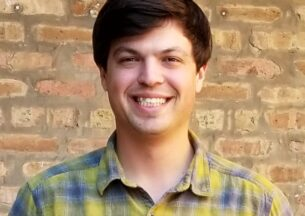Contact Info
Phone
773-702-2358
Office
Crerar 203

## Research

My research interest is in theoretical computer science (TCS) and discrete mathematics. TCS is in the overlap of mathematics and computer science; it deals with the rigorous analysis of algorithms and mathematical models of computation. Specifically, I have worked in the complexity theory of Boolean functions and in algorithmic coding theory. An overarching theme of my work has been the application of group theory to algorithms and complexity theory.

I am often drawn to problems with a discrete, symmetrical structure that is simple to describe but hard to analyze.

### Theory

The mathematical foundations of computation, including algorithm design, complexity and logic

## News & EventsPast Event

Sep 03, 2019